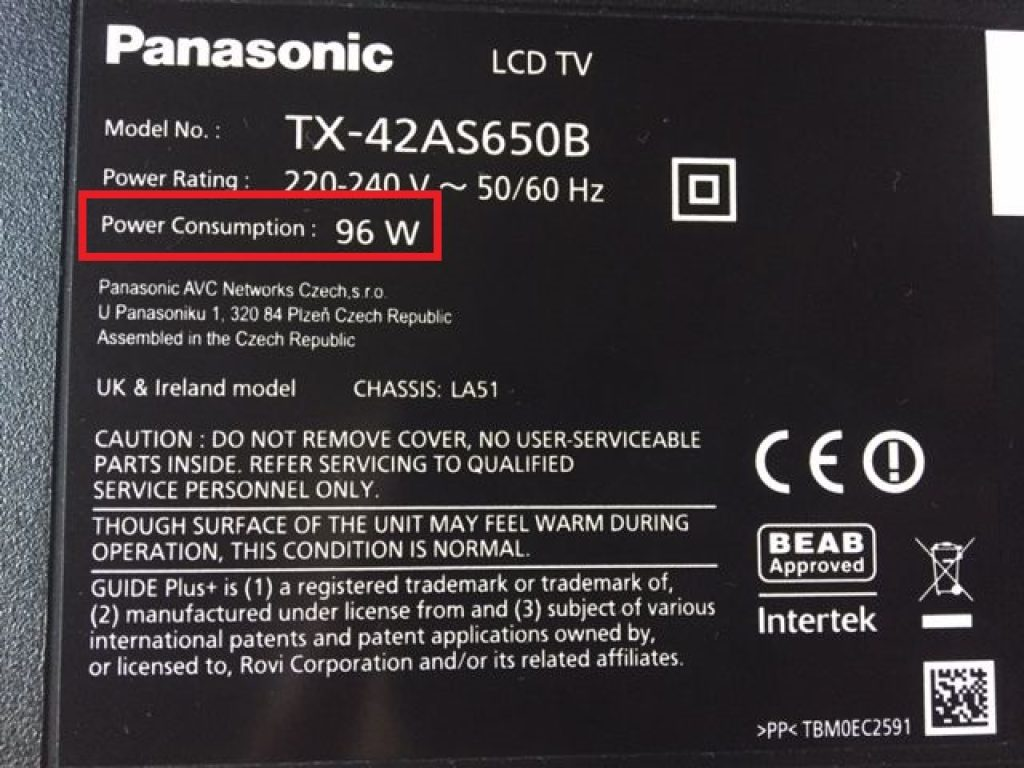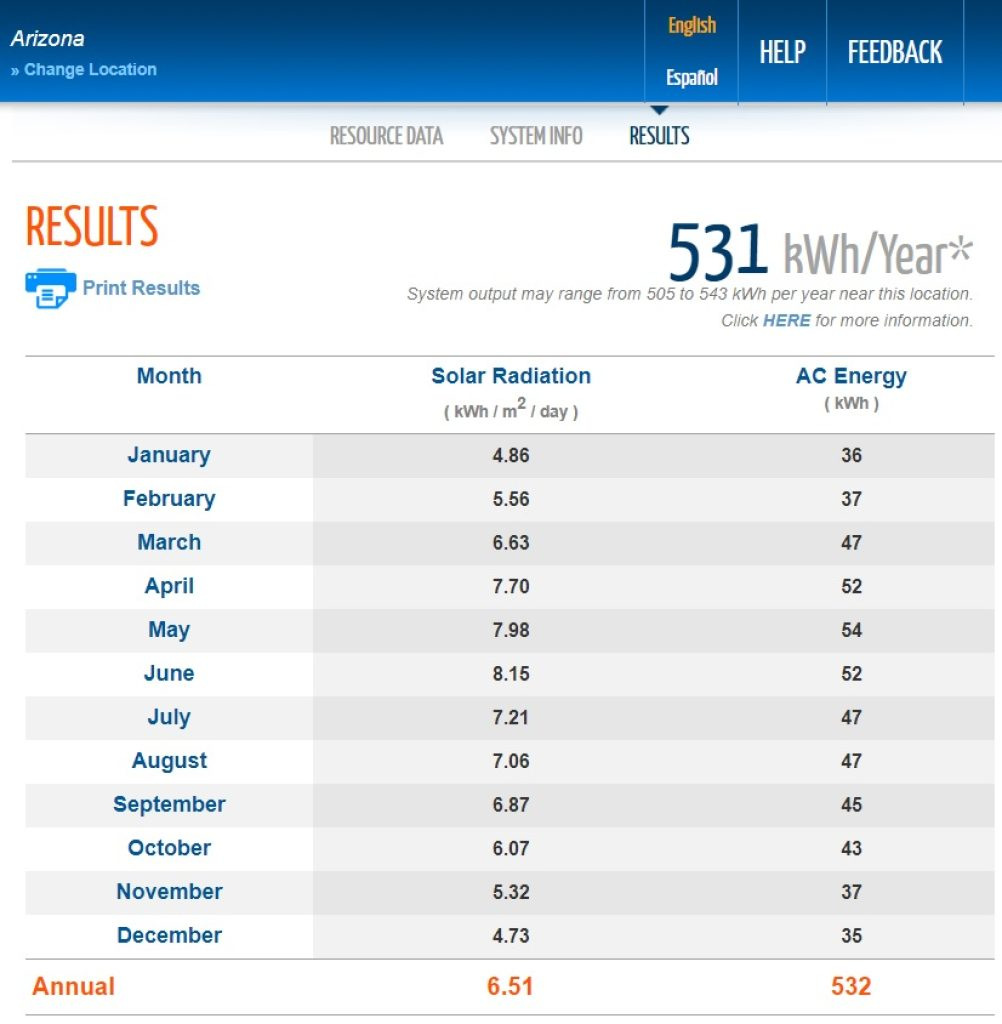# What can a 300-watt solar panel run?

A 300-watt solar panel is a popular choice for residential solar power systems — it can generate a relatively high amount of energy, enough to run many of your typical house appliances.

But let’s get specific here. What kind of appliances can you expect to run with a 300-watt solar panel?

Let’s find out!

Foreword
Climatebiz experts design, research, fact-check & edit all work meticulously.

### Affiliate Disclaimer

Climatebiz is reader-supported. We may earn an affiliate commission when you buy through links on our site.

## What can a 300-watt solar panel run?

A 300-watt solar panel is at the upper end of what you could expect from an average-size solar panel. It can generate a significant amount of energy to run various house appliances.

So, what sort of appliances can you expect it to run?

The daily average energy output of a 300W solar panel allows it to power any small portable device. Some examples include:

• Laptop chargers.
• Phone chargers.
• Camera/drone chargers.
• Tablets.
• Fans.
• Router.
• Lamps.

Now, what about the more “power-hungry” appliances? Can they power refrigerators, ACs, freezers, microwaves, and hairdryers?

To answer these questions, we need to look closely at how much power a 300W solar panel can produce and how much power these appliances require.

### How to determine what a 300W solar panel can run

To determine whether your 300-watt solar panel can run a particular appliance, you need to establish how much energy (in Wh) your panel produces and how much power (in W) your appliance consumes.Typically, you’ll find a label at the back or bottom of your appliance containing the wattage/power consumption information. If you can’t find its power rating, you can multiply the voltage by the current to find the power consumption, like so: Power (W) = Voltage (V) x Current (A)

#### Example

On average, your 300-Watt solar panel produces 1000Wh (1kWh) daily.

Can you run a TV with a power consumption of 100W with this panel? The answer is yes. In fact, you’d be able to power your TV for about 10 hours. Here’s how we know:

Energy (Wh) = Power (W) x Runtime (h) Power (W) = Energy (Wh) / Runtime (h)
Runtime (h) = Energy (Wh) / Power (W)

Therefore,

Runtime (h) = 1000 Wh / 100 W = 10 hours

Related reading: kW vs. kWh (An Energy Expert Explains The Difference)

## 300-watt solar panel power chart

The following table represents the appliances a 300-watt solar panel can run.

The table considers the average daily energy production of a 300W solar panel (1.1kWh) based on the average daily peak sun hours in the U.S. and the average wattage of common house appliances.

## How much energy does a 300-watt solar panel produce?

Not all 300-watt solar panels will produce the same energy output. This is because solar panel output depends on the amount of solar irradiance that reaches the panel, which in turn, varies according to several factors, including:

• Location.
• Time of year.
• Tilt/angle.
• Solar panel efficiency.

Using the average peak sun hours for each state, we can estimate that the energy generated by a 300W solar panel in the U.S. varies from 0.85 kWh to 1.37 kWh, depending on your location.

Therefore, the daily average energy production of a 300-watt solar panel in the U.S. is 1.1 kWh per day or 401 kWh per year.

But how can you estimate solar panel energy production?

### 1. Using The Climatebiz Solar Calculator

You can use our solar calculator to estimate how much energy a 300-watt solar panel will produce at your location.

#### Example

We estimated the energy production of a 300W solar panel in Nevada. Our solar calculator indicated that it produces around 545 kWh per year. This equates to a daily average of 1.49 kWh.

Related reading: Solar Panel Energy Production (State-by-state)

### 2. Using Peak Sun Hours (PSH)

You can find the average peak sun hours (PSH) for your state (see our list) and apply it to the following formula:

Solar Panel Energy Output (Wh) = Solar Panel Wattage (W) x Number of PSH (h) x 80%

Note to our readers: We multiply the result by 80% to account for system inefficiencies. In real-life conditions, a 300W doesn’t produce precisely 300W per hour of sunlight. This figure is always a bit less due to unfavorable conditions, such as shading, non-optimal angles, temperature, etc.

#### Example

The average PSH in California is 5.2 hours. Therefore, a 300W panel will produce a daily average of 1,248kWh:

Solar Panel Output (Wh) = 300 W x 5.2 h x 80% = 1248 Wh = 1,248 kWh

For New Jersey, however, the average daily PSH is 4 hours. Therefore, a 300W panel will produce a daily average of 9,6 kWh.

Solar Panel Output (Wh) = 300 W x 4 h x 80% = 960 Wh = 9,6 kWh

### 3. Using PVWatts

PVWatts is an online calculator developed by the National Renewable Energy Laboratory (NREL) that helps you estimate solar energy production.

To use it, fill in your information, such as your location, solar panel wattage, etc., and the calculator will show you how much energy your system can produce each month.

We used PVWatts to estimate the energy production of a 300-watt system in Arizona. Here are the results:PVWatts results for the average energy output of a 300 W solar panel in Arizona.Source: Climatebiz

As you can see, solar radiation can vary significantly throughout the year. In May, the average energy production was 54kWh, equal to 1.74 kWh per day. In January, however, energy production was 1.16 kWh per day.

## Final Thoughts

A 300-Watt solar panel can generate 1.1 kWh daily (in the U.S.), enough to run many house appliances and portable devices.

However, it’s important to remember that this number can vary significantly according to your location and the time of year.

For a more accurate number, you can apply your location’s average daily peak sun hours (see our list) to the formula previously shown in this article. Alternatively, you can use an online solar energy calculator, such as the PVWatts, developed by the NREL or our Climatebiz solar calculator.

Knowing how much energy your 300-watt solar panel can produce daily and how much power your appliances consume, you can figure out if the energy is enough to run your appliances and how long they will run.

Finally, a 300W solar panel is a fantastic option for residential applications and those who need to generate power on the road. For the latter option, you can find lightweight 300W solar panel models in compact sizes, such as the Bluetti model, the Goal Zero, and the AlphaESS.

Tags:Ana Lejtman

Ana is a Research Chemist with a strong background in Environmental chemistry. She's deeply interested in how chemistry can be applied to the development of green technologies. Her passion for sustainable chemistry and the environment led her to join the Climatebiz team, writing informative articles on renewable energy/green technologies related topics.